DAY 29
0

[Day-29] 增強式學習 (DQN) - 股票操作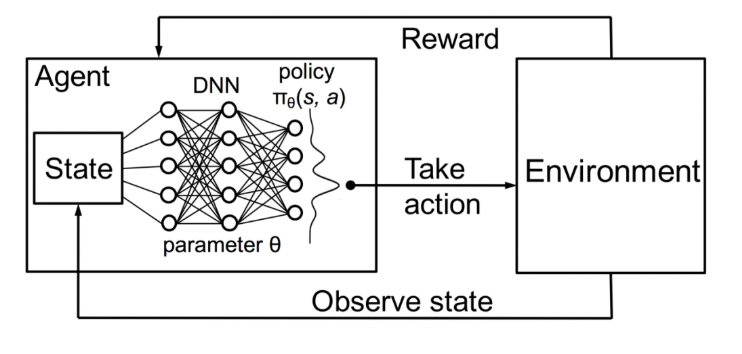source

DQN - 更新公式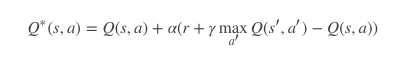DQN - Loss Function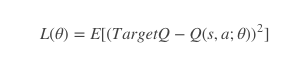stock_name = "TSM"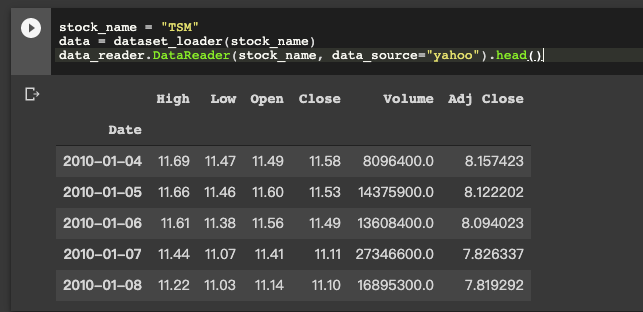def state_creator(data, timestep, window_size):

starting_id = timestep - window_size + 1
if starting_id >= 0:
windowed_data = data[starting_id:timestep+1]
else:
windowed_data = - starting_id * [data] + list(data[0:timestep+1])
state = []
for i in range(window_size - 1):
state.append(sigmoid(windowed_data[i+1] - windowed_data[i]))

return np.array([state])

Model 的部分，因為我們是操作股票，所以並非和一般玩遊戲可能使用CNN。

def model_dnn(self):

model = tf.keras.models.Sequential()

return model

(* action_num = 可操作數量 ， Ex: 買進、賣出、等候)

def batch_train(self, batch_size):
batch = []
# get pervious action
for i in range(len(self.memory) - batch_size + 1, len(self.memory)):
batch.append(self.memory[i])
for state, action, reward, next_state, done in batch:
reward = reward
if not done:
reward = reward + self.gamma * np.amax(self.model.predict(next_state))
target = self.model.predict(state)
target[action] = reward

self.model.fit(state, target, epochs=1, verbose=0)

elif action == 2 and len(trader.inventory) > 0: #Selling

reward = max(data[t] - buy_price, 0)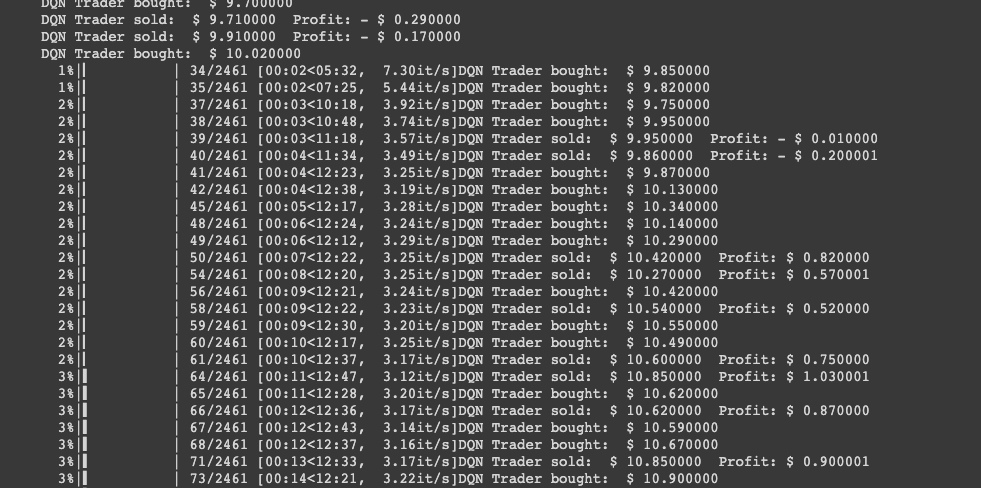一日一梗圖:source

DQN

0